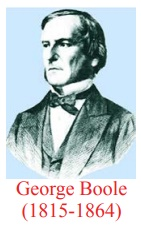Home | | Maths 12th Std | Mathematical Logic

# Mathematical Logic

Generally Logic is the study of valid reasoning. But mathematical logic allows us to represent knowledge in a precise mathematical way and it also allows us to make valid inferences using a set of precise rules.

Mathematical Logic

George Boole was a self-taught English Mathematician, Philosopher and Logician. His results on Boolean Algebra involving the binary numbers play an important role in various fields, particularly more in computer applications. He introduced the idea of Symbolic Logic and contributed a lot of results to the fast development of Mathematical Logic.The reputed Greek philosopher Aristotle (384-322BC(BCE)) wrote the first book on logic. The famous German philosopher and mathematician Gottfried Leibnitz of 17th century framed the idea of using symbols in Logic. Later this idea was realized by George Boole and Augustus de Morgan in 19th century. George Boole established the fact that logic is very much related to mathematics by linking logic, symbols, and algebra together. Mathematical Logic was developed in the late 19th and early 20th centuries.

In 1930 the researchers noticed (Neumann’s statement in his death bed: 0 and 1 are going to rule the world) that the binary numbers 0 and 1 could be used to analyze electrical circuits and thus used to design electronic computers. Today digital computers and electronic circuits are designed to implement this binary arithmetic. We study Mathematical Logic as the language and deductive system of Mathematics and Computer Science.

Generally Logic is the study of valid reasoning. But mathematical logic allows us to represent knowledge in a precise mathematical way and it also allows us to make valid inferences using a set of precise rules. It is regarded as a powerful tool for computer science because it is mainly used to verify the correctness of programs.

Tags : Discrete Mathematics | Mathematics , 12th Maths : UNIT 12 : Discrete Mathematics
Study Material, Lecturing Notes, Assignment, Reference, Wiki description explanation, brief detail
12th Maths : UNIT 12 : Discrete Mathematics : Mathematical Logic | Discrete Mathematics | Mathematics